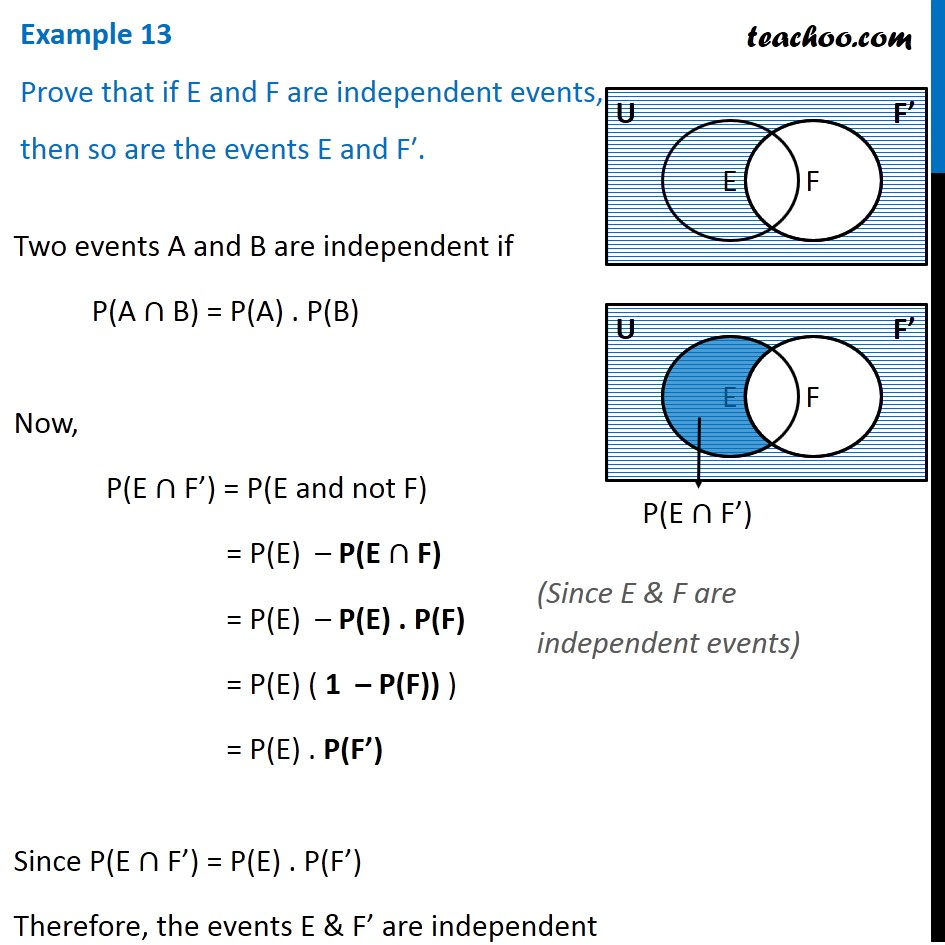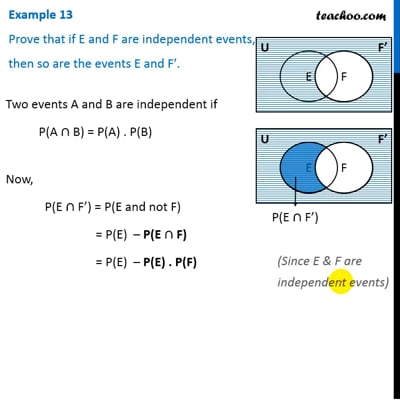Examples

Chapter 13 Class 12 Probability
Serial order wiseThis video is only available for Teachoo black users

Solve all your doubts with Teachoo Black (new monthly pack available now!)

### Transcript

Example 13 Prove that if E and F are independent events, then so are the events E and F′. Two events A and B are independent if P(A ∩ B) = P(A) . P(B) Now, P(E ∩ F’) = P(E and not F) = P(E) – P(E ∩ F) = P(E) – P(E) . P(F) = P(E) ( 1 – P(F)) ) = P(E) . P(F’) Since P(E ∩ F’) = P(E) . P(F’) Therefore, the events E & F’ are independent